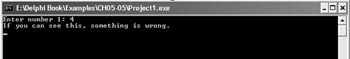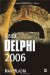Creating Functions

When you create a function, you have to define at least two things: the name of the function and the data type of the result. For instance, this is a function that returns a string value:

function ReturnString: string; begin end;

The function header tells Delphi that the function returns a string, but it actually returns nothing. To return a value from a function, we have to assign a value to a special variable called Result. The variable Result is a special variable that is created implicitly in every function. So, to return the string "Delphi" from the function, we have to write the following:

function ReturnString: string; begin   Result := 'Delphi'; end;

Since this function always returns the same value, it is also known as a constant function. Although constant functions might not seem very useful, they actually are, especially in larger projects.

A constant function can be used instead of a variable or a constant in an application to provide a consistent way of reading a value. As the complexity of the application grows, you may have to add more code to the function, perhaps to perform range checking or something similar. In this case, you'll only have to change the implementation of the function, and since you've been using the function from the start, everything works great. If you've used a variable or a constant, you'll waste time by finding and replacing the variable with the function call.

If you finish the project, and the constant function still returns only this constant value, you can replace the constant function with a constant value of the same name to optimize application performance. If you use Delphi 2005 or a newer Delphi version exclusively, a better solution would be to mark the constant function with the inline directive. This directive is described in more detail later in this chapter.

Short-circuit Evaluation

Short-circuit evaluation is the default Boolean evaluation mode that results in faster code execution than complete Boolean evaluation. In the vast majority of cases, short-circuit evaluation works perfectly, but you can get into trouble when you call functions inside the if-then statement that do more than just return a value. The following example has a subtle bug caused by short-circuit evaluation.

Listing 5-5: Short-circuit evaluation bugprogram Project1; {\$APPTYPE CONSOLE} uses   SysUtils; var   x: Integer;   FunctionCall: Boolean; function MakeTrue: string; begin   FunctionCall := True;   Result := 'Hello'; end; begin   FunctionCall := False;   Write('Enter number 1: ');   ReadLn(x);   if (x = 1) and (MakeTrue = 'Hello') then     WriteLn('Everything works fine.');   if FunctionCall = False then     WriteLn('If you can see this, something is wrong.');   ReadLn; end.This example features a really simple function called MakeTrue. The purpose of the MakeTrue function is to return the string "Hello" and to change the value of the FunctionCall variable to True.

When the application starts, it initializes the FunctionCall variable to False. It then asks the user to enter the number 1 and writes the user-entered value into variable x. The problem lies in the if-then statement that tests the x variable. If the user entered 1, the first part of the if-then statement evaluates to True, and the function MakeTrue is called in the evaluation process of the second part of the if-then statement. The function changes the FunctionCall variable to True and the application works as planned. In this case, you never see the message "If you can see this, something is wrong."

If the user enters anything but 1, the first part of the if-then statement fails. When the first part of the if-then statement fails, the entire statement fails, and the MakeTrue function is never called because the second part of the if-then statement is never evaluated. The FunctionCall variable remains False, and the error message that should never be displayed is displayed as shown in Figure 5-1.Figure 5-1: Short-circuit evaluation bug

If you only have one function call in the if-then statement, you can solve this problem by testing the function result first:

if (MakeTrue = 'Hello') and (x = 1) then   WriteLn('Everything works fine.');

Another solution is to temporarily enable complete Boolean evaluation. You should do this if you have multiple function calls that affect the application globally:

{\$B+} if (x = 1) and (MakeTrue = 'Hello') then   WriteLn('Everything works fine.'); {\$B-}Inside Delphi 2006 (Wordware Delphi Developers Library)
ISBN: 1598220039
EAN: 2147483647
Year: 2004
Pages: 212